# Some Uniform Polyhedra

Home

Regular Polyhedra

Stellations of the Icosahedron

Regular polyhedra havethe same regular polygon for all faces, and the same arrangement of these polygons at every vertex. For example, the cube has squares for all faces and three squares meet at every vertex. Uniform polyhedra keep the requirement for all vertices to be the same and all their faces have to be regular polygons, but the faces don't have to be all the same polygon.

There are an infinite number of uniform polyhedra if prisms and antiprisms are counted, but without the infinite classes there are 75 or 76, depending on the criteria used. This page just shows pictures of the ones which have the vertices of the dodecahedron or the icosidodecahedron, which are among the most pleasing in appearance.

Parts of the names of the polyhedra below have the following meanings:
• "Dodec" indicates that the polyhedron has faces in the twelve planes of a dodecahedron. These faces will be five-sided: either pentagons or pentagrams.
• "Icos" indicates that the polyhedron has faces in the twenty planes of an icosahedron. These faces will be equilateral triangles.
• "Hemi" indicates that the following number of faces are halved. Both the dodecahedron and the icosehedron have opposite face planes making parallel pairs. Replacing each pair by one plane through the centre gives the "hemi" arrangement. "Hemidodec" faces are ten-sided rather than five-sided and "hemicos" faces are hexagonal rather than triangular.
• "Ditrigonal" indicates that there are six faces meeting at a vertex; there are two types of face and they alternate around the vertex.
• "Great" and "small" distinguish different polyhedra which otherwise have the same names for their symmetries. The "great" polyhedron has faces deeper inside, nearer its centre.

Click on the images below to see animations of the polyhedra rotating.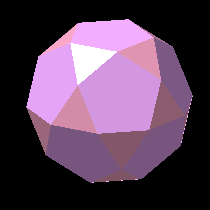The icosidodecahedron has 12 pentagonal faces in the planes of the dodecahedron and also 20 triangular faces in the planes of the icosahedron. It can be formed by slicing off vertex pieces from either the icosahedron or the dodecahedron. It is actually the volume interior to both when they are placed as a reciprocal pair.The small ditrigonal icosidodecahedron still has 20 triangular faces, but instead of pentagons it has pentagram (five-pointed star) faces. The star faces cause the triangular faces to intersect. Intersecting faces are a feature of all the following uniform polyhedra.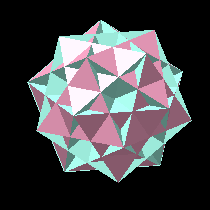The great ditrigonal icosidodecahedron has 20 triangular and 12 pentagon faces as suggested by the name "icosidodecahedron", but it differs from the basic icosidodecahedron because the pentagon faces are deep within the structure. Three triangles and three pentagons alternate around each vertex. We can see the edges of the regular compound of five cubes, suggesting relationships between the two configurations.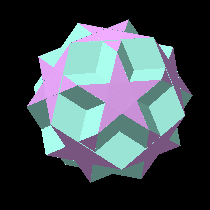The dodecadodecahedron has two sets of faces with the symmetry of the dodecahedron. These are outer pentagram faces and deeper pentagon faces. The 24 face planes lie in 6 sets, each consisting of 4 parallel planes.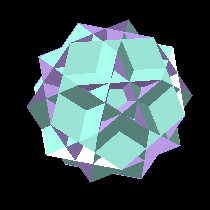The great dodecahemicosahedron has 12 pentagon faces in the dodecahedral arrangement. These are joined by 10 (not 20; hence "hemi") hexagon faces passing through the centre of the solid.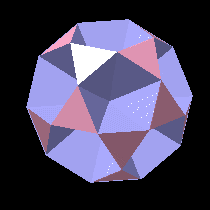The small icosihemidodecahedron can be recognised as having the 20 triangular faces of the icosidodecahedron, but the pentagon faces are missing. Instead, the triangular faces are joined by 6 decagon (ten-sided polygon) faces. Each decagon passes through the centre of the solid and divides it into two congruent halves.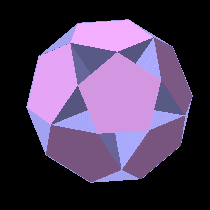The small dodecahemidodecahedron is a similar solid using the pentagon faces of the icosidodecahedron and the same 6 decagon faces through the centre. This polyhedron and the previous one together enclose the same volume as the icosidodecahedron.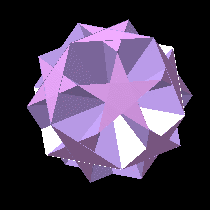The small dodecahemicosahedron has 12 pentagram faces in the dodecahedral planes and the 10 through-centre hexagon faces of the hemicosahedron.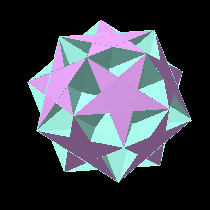The ditrigonal dodecadodecahedron has 12 pentagram faces, but now they are joined by 12 pentagon faces deep in the structure. Three of each kind of face alternate around each vertex. As the solid rotates, deep cavities between the pentagram arms are seen.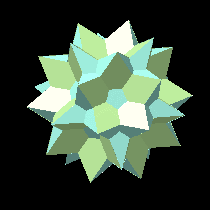The great icosidodecahedron has 20 triangular faces and 12 pentagram faces. Now the pentagram faces which are deep in the structure too.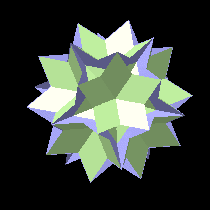The great icosihemidodecahedron has 20 triangular faces and 6 faces in the planes of a dodecahedron. However, the 6 faces are not decagons but decagrams: 10-pointed star polygons. These decagrams enclose their centres three times rather than twice as for a pentagram. They pass through the centre of the solid and only their outer areas are visible, inside the cups formed by groups of six triangle points.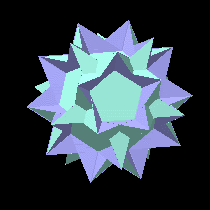The great dodecahemidodecahedron is the only polyhedron on this page which has all star-polygon faces: it has 12 pentagrams and 6 decagrams.

Home